Get our FREE VBA eBook of the 30 most useful Excel VBA macros.

Automate Excel so that you can save time and stop doing the jobs a trained monkey could do.

# Power Query – Running TotalIn this post, I want to cover a common reporting requirement, which is a Power Query running total.  I have previously covered a similar topic in a post about running totals in tables; however, those methods don’t work in Power Query.  So, we’ll need to find an alternative approach.

Both Tables and Power Query were designed with row context.  This basically means using the structured referencing systems, a formula can only retrieve values from a column in the same row; (i.e., there is no simple way to refer to the rows above or below).  With tables, we can resolve the running total issue using formulas that return ranges such as INDEX or OFFSET.  However, Power Query doesn’t have these or even equivalent functions.  Therefore, to solve this problem for Power Query, we are turning to the M code list functions.

I recommend you download the example file for this post.  Then you’ll be able to work along with examples and see the solution in action, plus the file will be useful for future reference.

Contents

In the sections below, we’ll look at how the two list functions work.  But if you want a quick answer, then follow the steps below.

Here is our example data, already loaded into Power Query.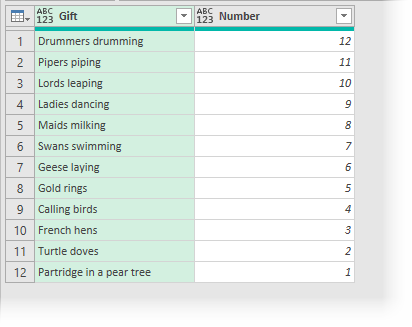The goal is to create a running total number of gifts received/given on the last day of the 12 Days of Christmas (based on the traditional song).

1. Add an Index column starting at 1 by clicking Add Column -> Index Column (drop-down) -> From 1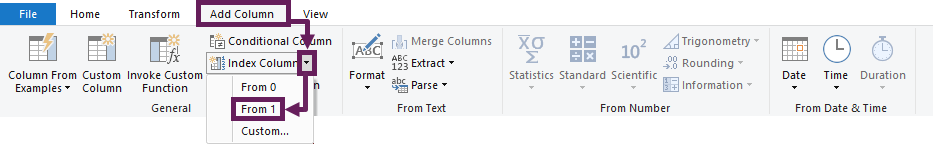2. Next, click Add Column -> Custom Column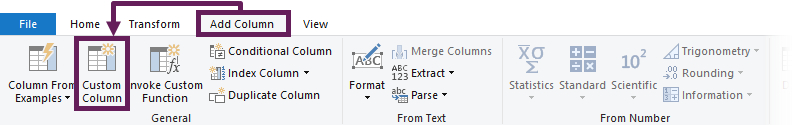3. In the Custom Column dialog box enter the following formula:
`=List.Sum(List.FirstN(#"Added Index"[Number],[Index]))`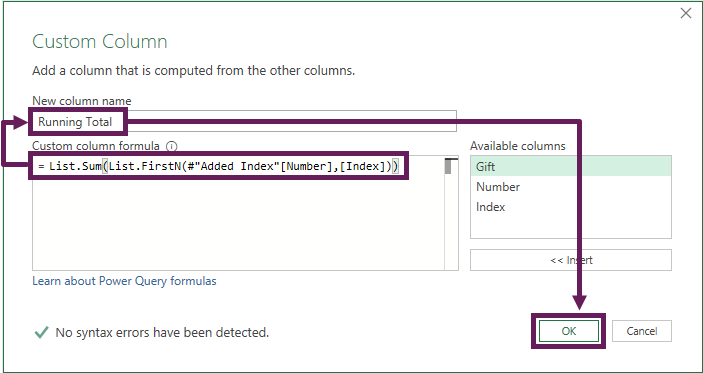4. Give the custom column a useful name, such as Running Total, then click OK.
5. The running total will now be included in the preview window.  You’re now free to delete the Index column if you wish.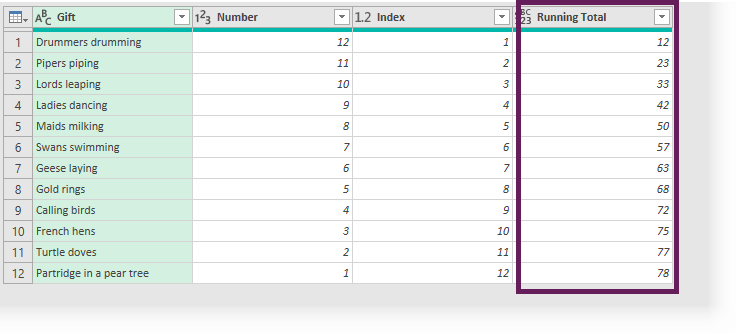For your scenario, you may need to change the following:

• Name of the column to use in the running total – in the example above it is the [Number] column, but will be different for your scenario.
• Name of the step which creates the Index column – in the example above it is the #”Added Index” step.  Look in the M code in the Advanced Editor if you’re unsure of the name of the step.
• Name of the Index column – in the example above it is called [Index]

You could leave now and be happy that you’ve found out how to do a Power Query running total.  But wouldn’t it be better to understand how this works? Why not hang around for a few minutes while I tell you :-).

## List Functions

The list functions are a group of Power Query M code functions that perform transformations on lists.  Lists can be either (a) a single column of data from a table or (b) a list of constants, such as {1,2,3} or {“cat”, “sat”, “mat”}

To create the running total, we use two list functions together:

Let’s look at each of these functions in more detail.

### List.FirstN

The List.FirstN function creates a list based on the first items of another list.  The first items can be defined as a specific number of items, or the first to meet a condition.

The format of the function is as follows:

`=List.FirstN(list as list, countOrCondition as any) as any`

Basic example #1:

In this example, we have a list of 5 constants, and the first 3 items are returned.

`=List.FirstN ({1,2,3,4,5}, 3)`
• list = {1,2,3,4,5}
• countOrCondition = 3

The result of the formula above would be {1,2,3}.

Basic example #2:

`=List.FirstN({1,2,3,4,5,3,2,1}, each _ <=3)`
• list = {1,2,3,4,5,4,3,2,1}
• countOrCondition = each _ <=3

The result of the formula above would be {1,2,3}

You might think it would return {1,2,3,2,1}, as they are all less than or equal to 3. However, as this is the List.FirstN function it only returns the results until the condition is false, and does not return any further results.

### List.Sum

The List.Sum function calculates the sum of the values in the list.

The format of the function is:

`=List.Sum(list as list, optional precision as nullable number)`

Basic example #3:

In this example, there is a list of 3 constants, which will be added together.

`=List.Sum({1,2,3})`
• list = {1,2,3}

The result of the formula above would be 6.

### Nesting List.FirstN into List.Sum

Just like standard Excel functions, with Power Query, we can nest a function inside another.

Basic example #4:

Combining example #1 and example #3 together, we get the following formula:

` =List.Sum(List.FirstN ({1,2,3,4,5}, 3))`

The result of the formula above is 6.  If you’re not sure how this works, then reread this section again; it is a crucial point.

If you want to learn more Power Query functions, you should check out Microsoft’s reference guide here: https://docs.microsoft.com/en-gb/powerquery-m/power-query-m-function-reference.## How it works

Now we understand how the functions work on a basic level; it’s time to turn back to our original running total example.

The source data, after creating the Index column, look like this: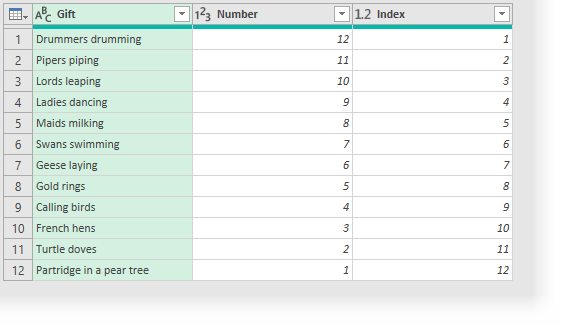First, we need to create a list with the correct items.  The formula to do that is this:

`=List.FirstN(#"Added Index"[Number],[Index])`
• list = #”Added Index”[Number]
This will take the step called #”Added Index” and use the column called Number as the basis of the list.  If you’re not sure of the M code name for the step, click View -> Advanced Editor.   The name of the step is the part we need (see highlight below).This part of the formula gives us a list of the values from the Number column of the data table {12,11,10,9,8,7,6,5,4,3,2,1}
• countOrCondition = [Index]
The first row has an Index of 1.  Therefore the List.FirstN function will return the first result from the list, which is {12}
The second row is an Index of 2 etc.  Therefore the function will return the first two items, {12,11}
The third row has an Index of 3.  Therefore the function will return the first 3 items, {12,11,10}
This continues for every row in the table

Then, we nest the function inside the List.Sum function:

`=List.Sum(List.FirstN(#"Added Index"[Number],[Index]))`

For the first row the result is 12, as the calculation would be:

• List.Sum({12})

The second row, the result is 23, as the calculation would be:

• List.Sum({12,11})

The third row, the result is 33, as the calculation would be:

• List.Sum({12,11,10})

The calculation is implemented for every row in the table.  By doing this, we have created a running total column in Power Query.

## Conclusion

The list functions are very powerful.  For Excel users, they can be challenging to understand, as the formula has to work for every row in the table, rather than a single cell.Get our FREE VBA eBook of the 30 most useful Excel VBA macros.

Automate Excel so that you can save time and stop doing the jobs a trained monkey could do.

Don’t forget:

If you’ve found this post useful, or if you have a better approach, then please leave a comment below.

Do you need help adapting this to your needs?

I’m guessing the examples in this post didn’t exactly meet your situation.  We all use Excel differently, so it’s impossible to write a post that will meet everybody’s needs.  By taking the time to understand the techniques and principles in this post (and elsewhere on this site) you should be able to adapt it to your needs.

But, if you’re still struggling you should:

1. Read other blogs, or watch YouTube videos on the same topic.  You will benefit much more by discovering your own solutions.
2. Ask the ‘Excel Ninja’ in your office.  It’s amazing what things other people know.
3. Ask a question in a forum like Mr Excel, or the Microsoft Answers Community.  Remember, the people on these forums are generally giving their time for free.  So take care to craft your question, make sure it’s clear and concise.  List all the things you’ve tried, and provide screenshots, code segments and example workbooks.
4. Use Excel Rescue, who are my consultancy partner.   They help by providing solutions to smaller Excel problems.

What next?
Don’t go yet, there is plenty more to learn on Excel Off The Grid.  Check out the latest posts:

## 3 thoughts on “Power Query – Running Total”

1.Areef Ali says:

Thanks – great explanation of List functions workings!
Keep up the good work

•Excel Off The Grid says:

Thanks Areef 🙂

2.sam says:

Thanks! I like the format of putting the answer up top first and then going into detail after.